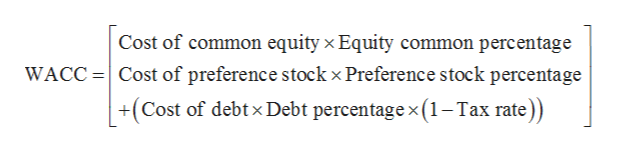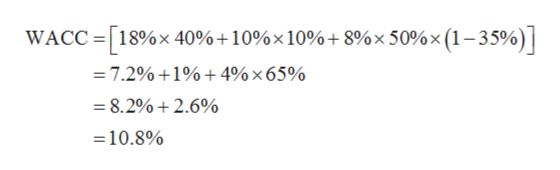# ​(Related to Checkpoint​ 14.1) ​ (Weighted average cost of​ capital)The target capital structure for QM Industries is 40 percent common​ stock, 10 percent preferred​ stock, and 50 percent debt. If the cost of common equity for the firm is 18 ​percent, the cost of preferred stock is 10 ​percent, the​ before-tax cost of debt is 8 percent, and the​ firm's tax rate is 35 ​percent, what is​ QM's weighted average cost of​ capital?QM's weighted average cost of capital is  ​%. ​(Round to three decimal​ places.)

Question
18 views

​(Related to Checkpoint​ 14.1) ​ (Weighted average cost of​ capital)

The target capital structure for QM Industries is 40 percent common​ stock, 10 percent preferred​ stock, and 50 percent debt. If the cost of common equity for the firm is 18 ​percent, the cost of preferred stock is 10 ​percent, the​ before-tax cost of debt is 8 percent, and the​ firm's tax rate is 35 ​percent, what is​ QM's weighted average cost of​ capital?

QM's weighted average cost of capital is  ​%. ​(Round to three decimal​ places.)

check_circle

Step 1

The formula to calculate the WACC is given below:help_outlineImage TranscriptioncloseCost of common equity x Equity common percentage WACCCost of preference stock x Preference stock percentage +(Cost of debtx Debt percentagex(1-Tax rate) fullscreen
Step 2

Substitute 40% for common equity percentage, 18% for cost of equity, 8% for cost of debt, 50% for debt perc...help_outlineImage TranscriptioncloseWACC 18%x 40%+10%x 10% + 8%x 50%x (1-35%) 7.2% +1%+ 4% x 65% = 8.2% +2.6% =10.8% fullscreen

### Want to see the full answer?

See Solution

#### Want to see this answer and more?

Solutions are written by subject experts who are available 24/7. Questions are typically answered within 1 hour.*

See Solution
*Response times may vary by subject and question.
Tagged in